Binary Search Tree(BST)

Binary search tree is a data structure that quickly allows us to maintain a sorted list of numbers.

• It is called a binary tree because each tree node has maximum of two children.
• It is called a search tree because it can be used to search for the presence of a number in O(log(n)) time.

The properties that separates a binary search tree from a regular binary tree is

1. All nodes of left subtree are less than root node
2. All nodes of right subtree are more than root node
3. Both subtrees of each node are also BSTs i.e. they have the above two properties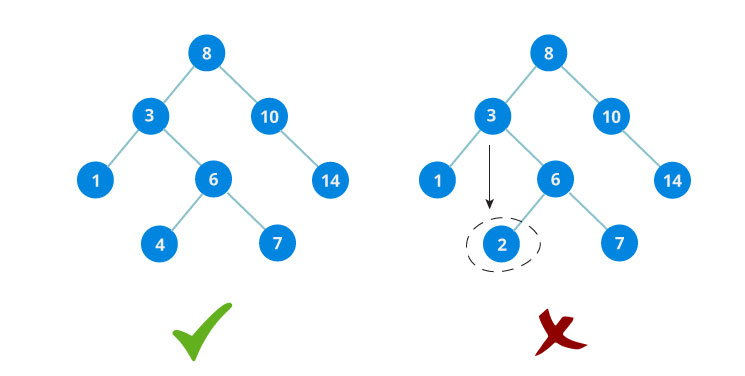The binary tree on the right isn't a binary search tree because the right subtree of the node "3" contains a value smaller that it.

There are two basic operations that you can perform on a binary search tree:

1. Check if number is present in binary search tree

The algorithm depends on the property of BST that if each left subtree has values below root and each right subtree has values above root.

If the value is below root, we can say for sure that the value is not in the right subtree; we need to only search in the left subtree and if the value is above root, we can say for sure that the value is not in the left subtree; we need to only search in the right subtree.

Algorithm:

If root == NULL
return NULL;
If number == root->data
return root->data;
If number < root->data
return search(root->left)
If number > root->data
return search(root->right)

Let us try to visualize this with a diagram.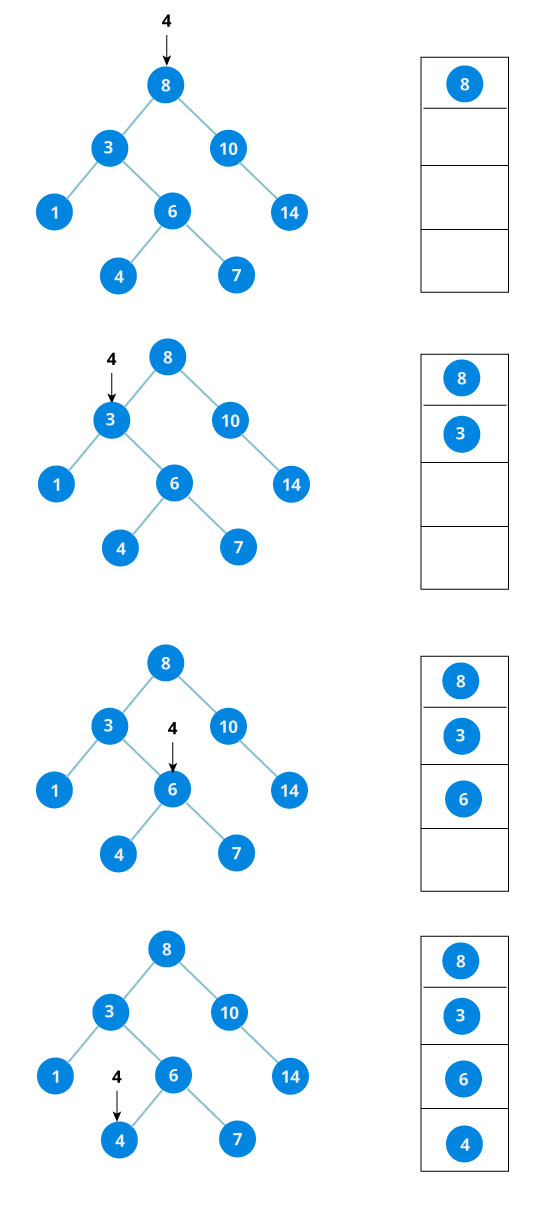If the value is found, we return the value so that it gets propogated in each recursion step as shown in the image below.

If you might have noticed, we have called return search(struct node*) four times. When we return either the new node or NULL, the value gets returned again and again until search(root) returns the final result.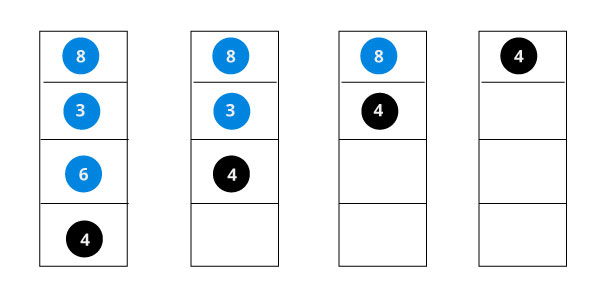If the value is not found, we eventually reach the left or right child of a leaf node which is NULL and it gets propagated and returned.

2. Insert value in Binary Search Tree(BST)

Inserting a value in the correct position is similar to searching because we try to maintain the rule that left subtree is lesser than root and right subtree is larger than root.

We keep going to either right subtree or left subtree depending on the value and when we reach a point left or right subtree is null, we put the new node there.

Algorithm:

If node == NULL
return createNode(data)
if (data < node->data)
node->left  = insert(node->left, data);
else if (data > node->data)
node->right = insert(node->right, data);
return node;

The algorithm isn't as simple as it looks. Let's try to visualize how we add a number to an existing BST.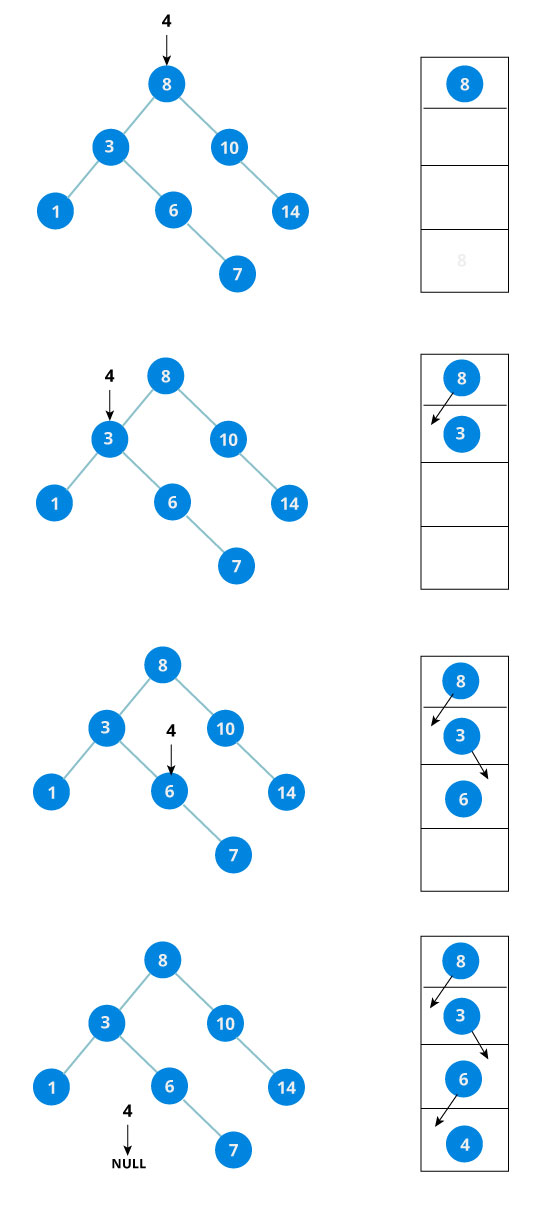We have attached the node but we still have to exit from the function without doing any damage to the rest of the tree. This is where the return node; at the end comes in handy. In the case of NULL, the newly created node is returned and attached to the parent node, otherwise the same node is returned without any change as we go up until we return to the root.

This makes sure that as we move back up the tree, the other node connections aren't changed.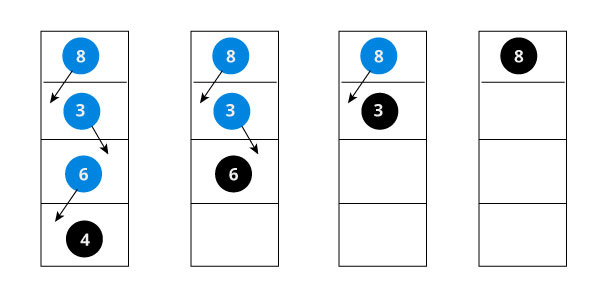The complete code for Binary Search Tree insertion and searching in C programming language is posted below:

#include<stdio.h>
#include<stdlib.h>

struct node
{
int data;
struct node* left;
struct node* right;
};

struct node* createNode(value){
struct node* newNode = malloc(sizeof(struct node));
newNode->data = value;
newNode->left = NULL;
newNode->right = NULL;

return newNode;
}

struct node* insert(struct node* root, int data)
{
if (root == NULL) return createNode(data);

if (data < root->data)
root->left  = insert(root->left, data);
else if (data > root->data)
root->right = insert(root->right, data);

return root;
}

void inorder(struct node* root){
if(root == NULL) return;
inorder(root->left);
printf("%d ->", root->data);
inorder(root->right);
}

int main(){
struct node *root = NULL;
root = insert(root, 8);
insert(root, 3);
insert(root, 1);
insert(root, 6);
insert(root, 7);
insert(root, 10);
insert(root, 14);
insert(root, 4);

inorder(root);
}

The output of the program will be

1 ->3 ->4 ->6 ->7 ->8 ->10 ->14 ->

Did you notice something?

The inorder traversal of a Binary Search Tree(BST) is a sorted list of numbers!